Courses

# Energy of a Satellite Class 11 Notes | EduRev

## Class 11 : Energy of a Satellite Class 11 Notes | EduRev

The document Energy of a Satellite Class 11 Notes | EduRev is a part of the Class 11 Course Physics Class 11.
All you need of Class 11 at this link: Class 11

6.2 Energies of a Satellite in a Circular Orbit

When there is a satellite revolving in a stable circular orbit of radius r around the earth, its speed is given by above equation. During its motion the kinetic energy of the satellite can be given as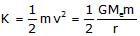...(1)

As gravitational force on satellite due to earth is the only force it experiences during motion, it has gravitational interaction energy in the field of earth, which is given as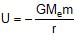...(2)

Thus the total energy of a satellite in an orbit of radius r can be given as

Total energy E = Kinetic energy + K Potential Energy U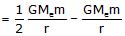or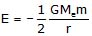...(3)

From equation (1), (2) and (3) we can see that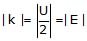The above relation in magnitude of total, kinetic and potential energies of a satelline is very useful in numerical problem so it is advised to keep this relation in mind while handing satellite problems related to energy.

Now to understand satellite and planetary motion in detail, we take few example.

Ex.9 Estimate the mass of the sun, assuming the orbit of the earth round the sun to be a circle. The distance between the sun and earth is 1.49 × 1011 m and G = 6.66 × 10-11 Nm2/kg2.

Sol. Here the revolving speed of earth can be given as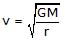[Orbital speed]

Where M is the mass of sun and r is the orbit radius of earth.

We known time period of earth around sun is T = 365 days, thus we have

T =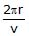or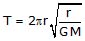or M =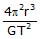=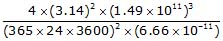= 1.972 × 1022 kg

Ex.10 If the earth be one-half of its present distance from the sun, how many days will be in one year ?

Sol. If orbit of earth's radius is R, in previous example we've discussed that time period is given as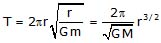If radius changes or r' =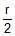, new time period becomes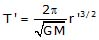From above equations, we have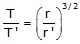or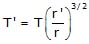=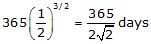Ex.11 A satellite revolving in a circular equatorial orbit of radius r = 2.00 × 104 km from west to east appear over a certain point at the equator every t = 11.6 hours. Using this data, calculate the mass of the earth. The gravitational constant is supposed to be known.

Sol. Here the absolute angular velocity of satellite is given by

ω = ωs + ωE

Where wE is the angular velocity of earth, which is from west to east.

or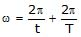[Where t = 11.6 hr. and T = 24 hr.]

From Kepler's III law, we have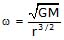Thus we have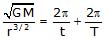or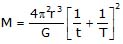= 6.0 × 1024 kg

Ex.12 A satellite of mass m is moving in a circular orbit of radius r. Calculate its angular momentum with respect to the centre of the orbit in terms of the mass of the earth.

Sol. The situation is shown in figure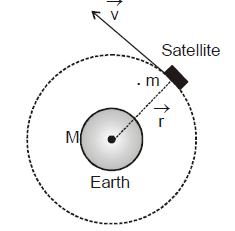The angular momentum of the satellite with respect to the centre of orbit is given by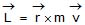Where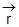is the position vector of satellite with respect to the centre of orbit and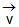is its velocity vector of satellite.

In case of circular orbit, the angle betweenandis 90°. Hence

L = m v r sin 90° = m v r ...(1)

The direction is perpendicular to the plane of the orbit.

We know orbital speed of satellite is...(2)

From equation (1) and (2), we get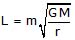⇒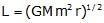Now we will understand the concept of double star system through an example.

Ex.13 In a double star, two stars of masses m1 and m2. distance d apart revolve about their common centre of mass under the influence of their mutual gravitational attraction. Find an expression for the period T in terms of masses m1, m2 and d. Find the ratio of their angular momenta about centre of mass and also the ratio of their kinetic energies.

Sol. The centre of mass of double star from mass m1 is given by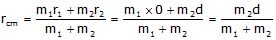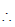Distance of centre of mass from m2 is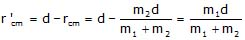Both the stars rotate around centre of mass in their own circular orbits with the same angular speed ω.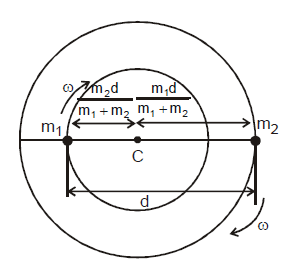the gravitational force acting on each star provides the necessary centripetal force. if we consider the rotation of mass m1, then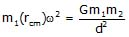or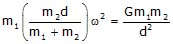This gives w =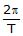=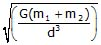or Period of revolution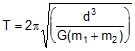Ratio of Angular Momenta is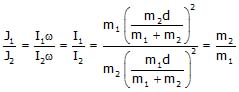Ratio of kinetic energies is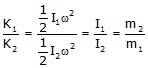7. Motion of a Satellite in Elliptical Path

Whenever a satellite is in a circular or elliptical path, these orbits are called bounded orbits as satellite is moving in an orbit bounded to earth. The bound nature of orbit means that the kinetic energy of satellite is not enough at any point in the orbit to take the satellite to infinity. In equation shown negative total energy of a revolving satellite shows its boundness to earth. Even when a body is in elliptical path around the earth, its total energy must be negative. Lets first discuss how a satellite or a body can be in elliptical path.

Consider a body (satellite) of mass m in a circular path of radius r around the earth as shown in figure. we've discussed that in circular path the net gravitational frame on body is exactly balancing the centrifugal force on it in radial direction relative to a rotating frame with the body.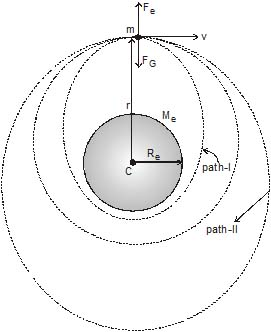If suddenly the velocity of body decreases then the centrifugal force on it becomes less then the gravitational force acting on it and due to this it can not continue in the circular orbit and will come inward from the circular orbit due to unbalanced force. Mathematical analysis shown that this path-I along which the body is now moving is an ellipse. The analytical calculations of the laws for this path is beyond the scope of this book. But it should be kept in mind that if velocity of a body at a distance r from earth's centre tangential to the circular orbit is less than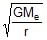then its path will be elliptical with earth centre at one of the foci of the ellipse.

Similarly if the speed of body exceedsthen it must move out of the circular path due to unbalancing of forces again but this time Fe > Fg. Due to this if speed of body is not increased by such a value that its kinetic energy can take the particle to infinity then it will follow in a bigger elliptical orbit as shown in figure in path-II, with earth's at one of the foci of the orbit.

In above case when speed of body was decreased and its value is lesser than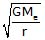and the speed is decreased to such a value that the elliptical orbit will intersect the earth's surface as shown in figure, then body will follow an arc of ellipse and will fall back to earth.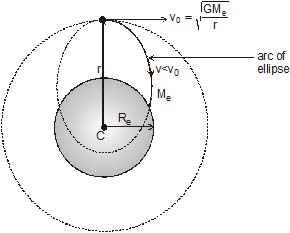7.2 Satellite Motion and Angular Momentum Conservation

We've discussed that when a body is in bounded orbit around a planet it can be in circular or elliptical path depending on its kinetic energy at the time of launching. Lets consider a case when a satellite is launched in an orbit around the earth.

A satellite S is first fired away from earth source in vertical direction to penetrate the earth's atmosphere. When it reaches point A, it is imparted a velocity in tangential direction to start its revolution around the earth in its orbit.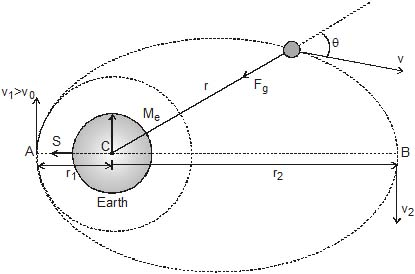This velocity is termed as insertion velocity, if the velocity imparted to satellite is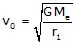then it starts following the circular path shown in figure. If velocity imparted is v1 > v0 then it will trace the elliptical path shown. During this motion the only force acting on satellite is the gravitational force due to earth which is acting along the line joining satellite and centre of earth.

As the force on satellite always passes through centre of earth during motion, we can say that on satellite there is no torque acting about centre of earth thus total angular momentum of satellite during orbital motion remains constant about earth's centre.

As no external force is involved for earth-satellite system, no external work is being done here so we can also state that total mechanical energy of system also remains conserved.

In the elliptical path of satellite shown in figure if r1 and r2 are the shortest distance (perigee) and farthest distance (appogee) of satellite from earth and at the points, velocities of satellite are v1 and v2 then we have according to conservation of angular momentum, the angular momentum of satellite at a general point is given as

L = mv1r1 = mv2r2 = mvr sinθ

During motion the total mechanical energy of satellite (kinetic potential) also remains conserved. Thus the total energy of satellite can be given as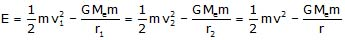Using the above relations in equation written above we can find velocities v1 and v2 of satellite at nearest and farthest locations in terms of r1 and r2.

Offer running on EduRev: Apply code STAYHOME200 to get INR 200 off on our premium plan EduRev Infinity!

## Physics Class 11

75 videos|312 docs|152 tests

,

,

,

,

,

,

,

,

,

,

,

,

,

,

,

,

,

,

,

,

,

;